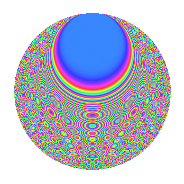# Properties

 Label 2100.2.blLevel 2100 Weight 2 Character orbit bl Rep. character $$\chi_{2100}(199,\cdot)$$ Character field $$\Q(\zeta_{6})$$ Dimension 288 Sturm bound 960

# Related objects

## Defining parameters

 Level: $$N$$ $$=$$ $$2100 = 2^{2} \cdot 3 \cdot 5^{2} \cdot 7$$ Weight: $$k$$ $$=$$ $$2$$ Character orbit: $$[\chi]$$ $$=$$ 2100.bl (of order $$6$$ and degree $$2$$) Character conductor: $$\operatorname{cond}(\chi)$$ $$=$$ $$140$$ Character field: $$\Q(\zeta_{6})$$ Sturm bound: $$960$$

## Dimensions

The following table gives the dimensions of various subspaces of $$M_{2}(2100, [\chi])$$.

Total New Old
Modular forms 1008 288 720
Cusp forms 912 288 624
Eisenstein series 96 0 96

## Trace form

 $$288q + 144q^{9} + O(q^{10})$$ $$288q + 144q^{9} - 20q^{14} + 16q^{16} + 28q^{44} - 8q^{46} + 24q^{49} + 68q^{56} + 96q^{64} + 72q^{66} + 56q^{74} - 144q^{81} + 8q^{84} + 4q^{86} + 72q^{94} + 60q^{96} + O(q^{100})$$

## Decomposition of $$S_{2}^{\mathrm{new}}(2100, [\chi])$$ into newform subspaces

The newforms in this space have not yet been added to the LMFDB.

## Decomposition of $$S_{2}^{\mathrm{old}}(2100, [\chi])$$ into lower level spaces

$$S_{2}^{\mathrm{old}}(2100, [\chi]) \cong$$ $$S_{2}^{\mathrm{new}}(140, [\chi])$$$$^{\oplus 4}$$$$\oplus$$$$S_{2}^{\mathrm{new}}(420, [\chi])$$$$^{\oplus 2}$$$$\oplus$$$$S_{2}^{\mathrm{new}}(700, [\chi])$$$$^{\oplus 2}$$

## Hecke characteristic polynomials

There are no characteristic polynomials of Hecke operators in the database